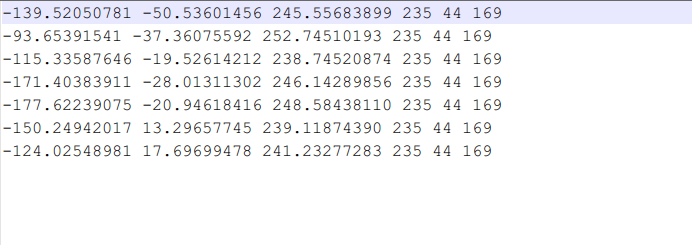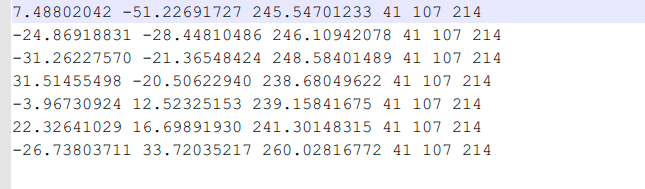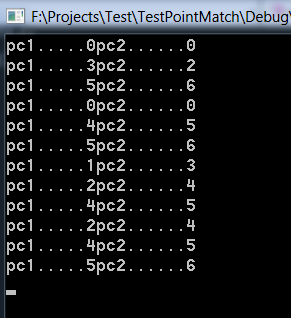• 利用C#编写的图像特征点的提取以及同名像点匹配程序
• 该文档介绍了基于Moravec算子的图像特征提取以及基于相关系数法的图像匹配的基本原理与编程实现方法，并附C#编写的程序源代码以及运行截图。
• 图像特征点提取与同名像点匹配_图像识别_同名匹配_源码.zip
• 基于c++编写实现大批量影像数据进行同名像点匹配计算
• 针对野外大视场、远距离、随机出现的特征相同多...多物体同名点提取以及实时匹配时间约为30 ms，满足相机25 frame/s的实时处理要求，较好地解决了超大三维空间中弧形坡面上随机出现的特征相同多物体同名点匹配问题。
• 针对目前三维激光扫描点云的同名标靶拼接大多采用手动且费时费力等问题,分别提出基于两和基于三点的同名标靶自动匹配算法。两种算法均可从含有不同数量的标靶的两站数据中快速寻找同名标靶。最后通过实验...
• 1.两个标靶数据 2.匹配结果 3.代码#include #include #include using namespace std; struct Point { int id; float x,y,z; Point(int _id,float _x,float _y,float _z):id
1.两个标靶数据2.匹配结果3.代码
#include <iostream>
#include <vector>
#include <math.h>
using namespace std;
struct Point
{
int id;
float x,y,z;
Point(int _id,float _x,float _y,float _z):id(_id),x(_x),y(_y),z(_z){}
};
struct Triangle
{
Point p1,p2,p3;
};
class PointCloud
{
public:
//void PointMatch(const PointCloud& pc1,const PointCloud &pc2);
vector<Point> pData;
int r,g,b;
};
{
if(filename==NULL)return false;
FILE *fp=fopen(filename,"r");
if (!fp)
{
return false;
}
char buffer;
int count=0;
while(!feof(fp))
{
fgets(buffer, 300, fp);
float x,y,z;
sscanf_s(buffer,"%f%f%f",&x,&y,&z);
Point p(count++,x,y,z);
pData.push_back(p);
}
pData.pop_back();
}
float calDis(const Point &p1,const Point &p2)
{
return sqrt((p2.x-p1.x)*(p2.x-p1.x)+(p2.y-p1.y)*(p2.y-p1.y)+(p2.z-p1.z)*(p2.z-p1.z));
}
void PointMatch(const PointCloud& pc1,const PointCloud &pc2)
{
for (int i=0;i<pc1.pData.size()-2;++i)
for(int j=i+1;j<pc1.pData.size()-1;++j)
for (int k=j+1;k<pc1.pData.size();++k)
{
Point p1=pc1.pData[i];
Point p2=pc1.pData[j];
Point p3=pc1.pData[k];
float s12=calDis(p1,p2);
float s13=calDis(p1,p3);
float s23=calDis(p2,p3);
for (int m=0;m<pc2.pData.size();++m)
for (int n=0;n<pc2.pData.size();++n)
for (int l=0;l<pc2.pData.size();++l)
{
if (n!=m&&m!=l&&n!=l)
{
Point _p1=pc2.pData[m];
Point _p2=pc2.pData[n];
Point _p3=pc2.pData[l];
float _s12=calDis(_p1,_p2);
float _s13=calDis(_p1,_p3);
float _s23=calDis(_p2,_p3);
if (fabs(s12-_s12)<0.45&&fabs(s13-_s13)<0.45&&fabs(s23-_s23)<0.45)
{
cout<<"pc1....."<<p1.id<<"pc2......"<<_p1.id<<endl;
cout<<"pc1....."<<p2.id<<"pc2......"<<_p2.id<<endl;
cout<<"pc1....."<<p3.id<<"pc2......"<<_p3.id<<endl;
}
}
}

}

}
int main()
{
char *file1="测试点云\\点云Mark0.txt";
char *file2="测试点云\\点云Mark1.txt";
PointCloud pc1,pc2;
PointMatch(pc1,pc2);
}
展开全文• 摄影测量手动提取立体像对同名点像素，相对定向结算，特征点提取，影像匹配同名点的python实现 ...（4）采用任意一种匹配算法，通过影像匹配取得同名像点，保存6-10个质量可靠、位置合理的同名像点坐标。重复上面
摄影测量手动提取立体像对同名点像素，相对定向解算，特征点提取，影像匹配同名点的python实现
0 写在前面
摄影测量课程布置的课程大作业，主要要求是： 独立完成一种相对定向的算法程序设计与开发。功能包括： （1）打开立体像对，手工屏幕量测像点坐标，转换成像平面坐标（o-xy），取得5个以上同名像点坐标并保存； （2）进行相对定向计算； （3）统计观测值的单位权中误差，并实施一种剔除粗差的处理。 （4）采用任意一种匹配算法，通过影像匹配取得同名像点，保存6-10个质量可靠、位置合理的同名像点坐标。重复上面的（2）（3）步骤； （5）比较手工量测和计算机自动匹配同名像点，两者对相对定向结果有无差别。使用的python库 numpy 操作数组和矩阵 cv2 操作图像 pandas 读写文件文中具体公式原理参照：摄影测量学第二版武汉大学出版社、误差理论与测量平差基础第三版武汉大学出版社。同时，代码也写了注释所有的文件代码都会上传，下载地址在文章最后
1 进行立体相对的手工屏幕量测
使用OpenCV，定义一个供setMouseCallback使用的回调函数mouse，这个回调函数在捕获到鼠标左键点击事件(cv2.EVENT_LBUTTONDOWN)时，获取点击的像素点坐标，并绘制一个实心的圆且显示出坐标。 具体实现参照： python+OpenCV交互图片获取鼠标单击坐标点（解决大图片显示不全问题）获取得到5对以上的同名点坐标，我选取了9对：
 uvs1 = [[275, 1566, 1], [3205, 1979, 2], [5729, 1748, 4], [6075, 3166, 5], [3933, 3189, 6], [566, 3121, 7], [326, 4469, 8], [3499, 4366, 9], [6110, 4535, 10]] #左片采样点像素坐标,其中3号点位为错误点
uvs2 = [[676, 315, 1], [3513, 521, 2], [6061, 56, 3], [6502, 1429, 4], [4325, 1659, 5], [1093, 1840, 6], [975, 3127, 7], [3979, 2832, 8], [6633, 2836, 9]] #右片采样点像素坐标[y,x,点号]

2 像素坐标与像平面、像空间、像空间辅助坐标的转换
像素点坐标转像平面坐标 坐标转换参照： 计算机视觉：相机成像原理：世界坐标系、相机坐标系、图像坐标系、像素坐标系之间的转换
xArrs1 = [] #存放得到的左片像点平面坐标[-x,-y,1] 因为左片在下，右片在上
xArrs2 = [] #存放得到的右片像点平面坐标

# 像素点坐标转像平面坐标
def pixelToImgPoint():
senserSize = [35.9, 24] #传感器尺寸 宽*高（mm）
pixelSize = [7360,4912] #像素尺寸 宽*高 （像素）
u0 = pixelSize / 2 #中心点像素横坐标
v0 = pixelSize / 2 #中心点像素横坐标
dx = senserSize / pixelSize #x轴1像素实际大小(mm)
dy= senserSize / pixelSize #y轴1像素实际大小
f = 35 #焦距（mm）
uvs1 = [[275, 1566, 1], [3205, 1979, 2], [5729, 1748, 4], [6075, 3166, 5], [3933, 3189, 6], [566, 3121, 7], [326, 4469, 8], [3499, 4366, 9], [6110, 4535, 10]] #左片采样点像素坐标,其中3号点位为错误点
uvs2 = [[676, 315, 1], [3513, 521, 2], [6061, 56, 3], [6502, 1429, 4], [4325, 1659, 5], [1093, 1840, 6], [975, 3127, 7], [3979, 2832, 8], [6633, 2836, 9]] #右片采样点像素坐标[y,x,点号]

A = np.array([[1/dx, 0, u0], [0, 1/dy, v0], [0, 0, 1]])
for arr1 in uvs1:
b = np.transpose(np.array([[arr1, arr1, 1]]))
x = np.linalg.solve(A, b)
xArrs1.append(x)
for arr2 in uvs2:
b = np.transpose(np.array([[arr2, arr2, 1]]))
x = np.linalg.solve(A, b)
xArrs2.append(x)


像平面转像空间辅助坐标
spaceArr1 = [] # 左像空间辅助坐标集合
spaceArr2 = [] # 右像空间辅助坐标集合
# 计算像空间辅助坐标
def computerPixelAssist(r, w, k):
f = 35.0  # 焦距（mm）
global spaceArr1,spaceArr2
spaceArr1, spaceArr2 = [], [] # 清空数组

if len(xArrs1) ==0 | len(xArrs2) == 0:
return

# 左相片做换为像空坐标
for arr1 in xArrs1:
# 交换x y轴 再取负
t = np.copy(arr1)
arr1 = -arr1
arr1 = -t

arr1 = -f
spaceArr1.append(arr1)

#计算右片旋转矩阵
# r, w, k = 0, 0, 0
a1 = np.cos(r)* np.cos(k) - np.sin(r) * np.sin(w) * np.sin(k)
a2 = -np.cos(r)* np.sin(k) - np.sin(r) * np.sin(w) * np.cos(k)
a3 = -np.sin(r)* np.cos(w)
b1 = np.cos(w)* np.sin(k)
b2 = np.cos(w)* np.cos(k)
b3 = -np.sin(w)
c1 = np.sin(r) * np.cos(k) + np.cos(r) * np.sin(w) * np.sin(k)
c2 = -np.sin(r) * np.sin(k) + np.cos(r) * np.sin(w) * np.cos(k)
c3 = np.cos(r) * np.cos(w)
R = np.array([[a1,b1,c1], [a2,b2,c2], [a3,b3,c3]]) #旋转矩阵

# 计算右片像空间辅助坐标
for arr2 in xArrs2:
# 交换x y轴 再取负
t = np.copy(arr2)
arr2 = -arr2
arr2 = -t

arr2 = -f
b = arr2
x = np.linalg.solve(R, b)

spaceArr2.append(x)


3 粗差检测、计算各个相对定向参数、相对定向解算、迭代计算、精度评定
采用连续法相对定向
相对定向、共面方程原理可参照： 摄测与CV 3: 共面方程，相对定向，绝对定向
粗差处理 数据探测法假设检验粗差，进行了粗差检验，并没有处理只是输出第几对像点存在粗差，粗差改正可后续添加 注：数据探测法假设检验参照误差理论与测量平差基础
def findCrossError():
global X
if (len(A) == 0) | (len(V) == 0):
return
q = 1 # 单位权中误差
Q = np.eye(9,9) # 9*9的协因数阵
Qvv = Q - (A * (A.T * A).I * A.T)
t = 0.05 # 正态分布的阿尔法值
ut = 1.96 # 正态分布表里的u0.025的限值
i = 0
for arr in V:
u = arr / (q * np.sqrt(Qvv[i,i]))
if np.abs(u) > ut:
print("第{}个点存在粗差,粗差为：{}".format(i,arr))
# 粗差处理
# X = np.zeros(shape=(5,1))# 舍去改正值
# print("已改正...")
else:
if i == len(V)-1: # 循环结束
print("未发现粗差...")
i += 1

各相对定向参数结算 解算参数和过程参照摄影测量学
X = 0 # A矩阵 L由观测值计算得到的q值 V五个参数的改正值 X真值
V = 0 # 存放改正值
A = 0 # 系数矩阵
def computerNewValue():
global X, V, A
L = 0
i = 0
while i < len(QArr):
x2, y2, z2, n1 = spaceArr2[i], spaceArr2[i], spaceArr2[i], N1Arr[i]
a1 = - (x2 * y2 / z2) * n1
a2 = - (z2 + y2 * y2 / z2) * n1
a3 = x2 * n1
a4 = Bx
a5 = - (y2 / z2) * Bx

a = np.array([[a1, a2, a3, a4, a5]])
if i == 0:
A = a
else:
A = np.row_stack((A, a))
i += 1

# print(len(A))
A = np.mat(A)  # 转换成矩阵
L = np.transpose(np.array([QArr]))
L = np.mat(L)

X = (A.T.dot(A)).I.dot(A.T).dot(L)  # A.T求转置 A.I求逆
V = (A.dot(X) - L)


入口函数、迭代计算和精度评定 由于得到的未知数改正值有时无法收敛到限值，未找到原因，故没有以改正值为迭代的收敛条件，而是以迭代次数为收敛条件
if __name__ == '__main__':
r, w, k = 0, 0, 0 # 起始值
u, v = 0, 0
# V = 0 #存放差值
rDiffi, wDiffi, kDiffi, uDiffi, vDiffi = 0,0,0,0,0  #各差值
pixelToImgPoint()  # 像素转像平面坐标

def computer():
global r,w,k,u,v,V
global rDiffi,wDiffi,kDiffi,uDiffi,vDiffi
computerPixelAssist(r, w, k) # 转像空间辅助坐标
computerParameter(u, v) # 计算各参数
computerNewValue() #计算新值
# 计算差值
# V = X - np.mat([[r,w,k,u,v]]).T
# print(X)
rDiffi = X[0, 0]   # 各个差值
wDiffi = X[1, 0]
kDiffi = X[2, 0]
uDiffi = X[3, 0]
vDiffi = X[4, 0]
computer()
findCrossError()

# 迭代计算，判断是否满足要求
limit = 0.08 # 限值
flag = True # 是否继续迭代的指示
num = 1 # 迭代次数

# r, w, k, u, v = V[0, 0], V[1, 0], V[2, 0], V[3, 0], V[4, 0]
# computer()
while num < 100: #迭代多少次停止
# if (np.abs(rDiffi) > limit) | (np.abs(wDiffi) > limit) | (np.abs(kDiffi) > limit) | (np.abs(uDiffi) > limit)| (np.abs(vDiffi) > limit): # 超出限值
print("已迭代：%d次..."%num)
r,w,k,u,v = rDiffi+r,wDiffi+w,kDiffi+k,uDiffi+u,vDiffi+ v # 参数改正
precision = np.sqrt((V.T.dot(V))/4)[0,0]
print("精度：{}毫米".format(precision))
computer()
num += 1
print("已迭代：%d次..." % num)
# else:
#     flag = False
#     break

print("================================================")
print("改正值：r:{}, w:{}, k:{}, u:{}, v:{}".format(rDiffi, wDiffi, kDiffi, uDiffi, vDiffi))
print("最终精度：{}".format(precision))
print("改正后的值：r:{}, w:{}, k:{}, u:{}, v:{}".format(r,w,k,u,v))

运行输出结果如下： 迭代次数、每次迭代后的精度、结果的改正值、结果的精度、相对定向的5参数 到此相对定向解算完成4 特征点提取
采用Moravex算子进行特征点提取，提取过程如下： 提取左影像的特征点、保存左影像特征点像素坐标、提取部分特征点进项右影像匹配、保存左右影像同名特征点
兴趣值计算 兴趣值计算比较耗时，需要计算接近7360*4912个的像素的兴趣值
# 计算感兴趣值 mat:灰度矩阵 fullSize窗口大小 x中心像元行号 y列号
def getInterestValue(mat, fullSize, x, y):
halfSize = fullSize // 2
i = 0
v1, v2,v3,v4 = 0,0,0,0
while i < halfSize * 2 :
v1 += np.power((int(mat[x-halfSize+i,y]) - int(mat[x-halfSize+i+1,y])),2)
v2 += np.power((int(mat[x-halfSize+i,y-halfSize+i]) - int(mat[x-halfSize+i+1,y-halfSize+i+1])),2)
v3 += np.power((int(mat[x,y-halfSize+i]) - int(mat[x,y-halfSize+i+1])),2)
v4 += np.power((int(mat[x-halfSize+i,y-halfSize-i]) - int(mat[x-halfSize+i+1,y-halfSize-i-1])),2)
i += 1
v = [v1, v2, v3, v4]
interest = np.min(v)
return interest

inputimagepath = "./image/left.JPG"
def gray_cvt(inputimagepath): # 入口函数
gray = cv2.cvtColor(img,cv2.COLOR_RGB2GRAY)# 灰度化 gray_cvt_image为灰度矩阵宽*高
# cv2.namedWindow('gray')  # 这行没啥用 控制显示图片窗口的名字
h, w = gray.shape
# Moravex算子
grayMat = np.mat(gray)
interests = np.zeros((h,w)) #兴趣值数组
fullSize = 9 #窗口大小
inhibitionsize = 17 #抑制局部最大的窗口大小
halfSize = fullSize // 2
halfInhiSize = inhibitionsize // 2
i, j  = halfSize, halfSize
sum = 0
while i < h-halfSize: # 行列数搞错了，计算结果出错 高度为行数
print("兴趣值计算到{}行".format(i+1))
j = halfSize # 找半天这个bug 还是太菜了
while j < w-halfSize:
interest = getInterestValue(grayMat,fullSize,i,j)
interests[i][j] = interest #bug 若直接拷贝原数组会部分赋值失败，可能是像素超过255便不赋值
sum += interest
j +=1
i+=1
print("所有兴趣值计算完毕...")


确定阈值、阈值过滤 滤值是由所有兴趣值的均值确定的 注：以下代码在在主函数内
	i, j = halfSize, halfSize
mean = sum / ((w-fullSize )* ( h-fullSize)) #阈值
print("阈值{}",format(mean))
while i < h-halfSize:
print("阈值过滤到{}行".format(i+1))
j = halfSize
while j < w-halfSize:
value = interests[i][j]
if value < mean:
interests[i][j] = 0
j +=1
i+=1
print("阈值过滤完毕...")

抑制局部最大
 # 抑制局部最大
i, j = halfSize, halfSize
while i < h-halfInhiSize:
print("抑制局部最大到{}行".format(i+1))
j = halfInhiSize
while j < w-halfInhiSize:
max = interests[i][j]
m, n  = 0,  0
while m < inhibitionsize:
n = 0
while n < inhibitionsize:
value = interests[i - halfInhiSize + m][j - halfInhiSize + n]
if  value < max:
interests[i - halfInhiSize + m][j - halfInhiSize + n] = 0
n += 1
m += 1
j +=1
i+=1
print("抑制局部非最大完毕...")

最后保存结果，结果为特征点的像素坐标和兴趣值
 # 保存结果
i, j = halfSize, halfSize
xs, ys, grayValue = [],[],[]
while i < h - halfSize:
j = halfSize
while j < w - halfSize:
value = interests[i][j]
if value > 0:
xs.append(j)
ys.append(x)
grayValue.append(value)
j += 1
i += 1
# 字典中的key值即为csv中列名
dataframe = pd.DataFrame({'X坐标': xs, 'Y坐标': ys ,'兴趣值': grayValue})
# 将DataFrame存储为csv,index表示是否显示行名，default=True
dataframe.to_csv("featurePoints.csv", index=False, sep=',')
print("所有特征点写入完毕...")
cv2.waitKey()#等待操作
cv2.destroyAllWindows()#关闭显示图像的窗口

运行结果，featurePoints.csv文件： 注：运行时没有写入兴趣值5 影像匹配
相关系数匹配特征点 思路是：读取左影像部分特征点，获得特征点特定大小（这里选取5*5像素）的窗口（目标窗口），获得右影像灰度矩阵（搜素窗口），右影像矩阵划分成目标窗口大小的矩阵，选定目标窗口逐个对搜素窗口划分后的小窗口进行系数计算，求出最大的系数，该系数对应的小窗口的中心像素即为匹配得到的右影像特征点坐标，最后写入文件
读取影像函数
def readImage(imageName):
gray = cv2.cvtColor(img, cv2.COLOR_RGB2GRAY)  # 灰度化 gray_cvt_image为灰度矩阵宽*高
# h, w = gray.shape
cv2.waitKey()  # 等待操作
cv2.destroyAllWindows()  # 关闭显示图像的窗口
return gray

读写文件函数
def readFile():
xys =  []

for i in range(10000,100000,5000): #间隔取点
x = df["X坐标"][i]
y = df["Y坐标"][i]
xys.append([x,y])
return xys

def writeFile(xs1,ys1,xs2,ys2):
dataframe = pd.DataFrame({'左影像X坐标': xs1, '左影像Y坐标': ys1,'匹配的右影像X坐标': xs2, '匹配的右影像Y坐标': ys2})
dataframe.to_csv("featurePoints_matching.csv", index=False, sep=',')
print("所有匹配特征点写入完毕...")

划分数组
# 划分数组
def divideArr(arr, rows, cloumns):
h, w = arr.shape
rowsNum, colNum = [],[]
i, j = 0,0
numRow = h // rows #分成几等行
numClo = w // cloumns #分成几等列
sigleXYS = [] # 单个搜索区窗口列表
# 拆分行
while  i < numRow * rows:
i += rows
rowsNum.append(i)
sigleX = np.split(arr, rowsNum, axis=0)
finallymat =  sigleX[len(sigleX)- 1]
gao1 ,kuan1 = finallymat.shape
if gao1 < rows:
del sigleX[len(sigleX)- 1]

t = 0
while t < len(sigleX):
# 拆分列
while j < numClo * cloumns:
j += cloumns
colNum.append(j)
sigleY = np.split(sigleX[t], colNum, axis=1)
finallymat2 = sigleY[len(sigleY) - 1]
gao1, kuan1 = finallymat2.shape
if kuan1 < cloumns:
del sigleY[len(sigleY) - 1]
sigleXYS.append(sigleY)
t += 1
return sigleXYS


4.求相关系数
# 求相关系数
def matching(mat1, mat2):
m,n  = mat1.shape
i,j = 0,0
fz1,fz2,fz3,fm1,fm2 = 0,0,0,0,0

while i <len(mat1):
j = 0
while j < len(mat2):
a,b = int(mat1[i][j]), int(mat2[i][j])
fz1 += a * b
fz2 += a
fz3 += b
fm1 += a *a
fm2 += b *b
j += 1
i += 1
fzp = fz1 - (1/(m* n)) *fz2 *fz3
fmp1 = fm1 - (1/(m*n))* fz2 *fz2
fmp2 = fm2 - (1/(m*n)* fz3 *fz3)
if fmp1* fmp2 == 0: #除数太小 python会看成0 报错
return 0
p = fzp / (np.sqrt(fmp1* fmp2)) #相关系数
return p


入口函数
def computerValue(g,g1):
h, w = g1.shape
m, n,k,l =5, 5,h, w # m n为目标窗口大小 k l 为搜索影像区域
r,c = m // 2, n//2
i,j = 0,0
sigleXYS = divideArr(g1,m, n) #982*1472
finalCol = len(sigleXYS)
finalRow = len(sigleXYS)

if len(xys) == 0:
print("读取文件失败...")

pipeiNum = 0
xbefore, ybefore, xmatch, ymatch = [], [],[],[]  # 匹配的坐标
for xy in xys:
x,y  = xy,xy
xbefore.append(x)
ybefore.append(y)
E = range(x-r, x+r+1)  # 定义列数
F = range(y-c, y+c+1)  # 定义行数
goalMat = g[F]  # 取出需要的行
goalMat = goalMat[:,E]  # 取出需要的列 X_3为目标矩阵

ps = [] # 相关系数集合

i = 0
while i < finalCol:
j = 0
while j < finalRow:
sigleMat = sigleXYS[i][j]
p = matching(goalMat,sigleMat)
ps.append(p)
j +=1
i += 1
pmax = np.max(ps)
maxIndex = ps.index(pmax)
goalRow = (maxIndex) // finalCol
goalCol = (maxIndex) % finalCol
goalRow = goalRow * m - c
goalCol = goalCol * n - r
xmatch.append(goalRow)
ymatch.append(goalCol)
pipeiNum += 1
print("已匹配:{}个像点".format(pipeiNum))

writeFile(xbefore,ybefore,xmatch,ymatch) #写入文件


featurePoints_matching.csv内容6 总结
相对定向的收敛条件无法满足，可能的原因是：1.相对定向计算错误，本人检查了几遍，没有发现错误之处。2. 手动选取的同名像点坐标不准确。3.应该不是粗差的问题，假设检验后提示未发现粗差。特征点的计算出的阈值太小，导致提取的特征点非常多，可按照经验值重新设置。影像匹配的目标窗口和搜索窗口有时候并没有重叠，导致匹配出的特征点不准确，同时，影像匹配后的同名点存在不准确的情况，有待进一步改正。计算效率和准确度都没有达到预期效果，不能算是成功完成，只能算是学习的过程。
本文的所有代码文件链接已上传至csdn，下载地址：
点击前往代码下载地址
展开全文python
• 主要介绍了c语言同名标靶自动匹配算法实现实例代码，分享了相关代码示例，小编觉得还是挺不错的，具有一定借鉴价值，需要的朋友可以参考下
• 课程第4章介绍了如何在两幅影像上匹配同名点匹配同名点是计算影像相对姿态的第一步。用光流、特征提取方法进行同名点匹配
课程的 YouTube 地址为：https://www.youtube.com/playlist?list=PLTBdjV_4f-EJn6udZ34tht9EVIW7lbeo4。视频评论区可以找到课程所使用课件与练习题的下载地址。
课程第4章介绍了如何在两幅影像上匹配同名点，匹配同名点是计算影像相对姿态的第一步。用光流、特征点提取方法进行同名点匹配。
1. 从影像到几何
影像呈现的是色彩与亮度，但 MVG 使用匹配点或线进行三维重建，所以从影像的色彩信息中提取点或线是传统 MVG 的第一步。
为什么说是“传统 MVG ”呢？因为在以前计算资源匮乏的时代，只能通过稀疏的点与线进行三维重建。当今计算资源丰富，内存能够存储大量的信息，可以将影像的每一个像素存入内存参与计算，这种方式属于“直接法”，在课程的后半部分会讨论到。
从影像中提取同名点有存在两大挑战：1. 非刚体变化，影像中出现的非刚体在两张影像对应的两个时间点上一些特征点完全消失，无法匹配；2. 非朗博面，存在镜面反射，在不同角度观察空间位置上的同一个点存在着亮度相差特别大的问题。
在同名点匹配可以分为两种情形：  1. 微小移动，意味着基线短，两张影像中同名特征点之间的距离短，容易通过小窗口找到，同名点的寻找方向也基本一致；  2. 长基线，相机移动很大，左影像的特征点有可能在右影像的任何位置上。
对于短基线的情形，使用光流法（Optical Flow）进行特征点匹配，该方法是 Lucas 与 Kanade 于1981年提出的。
在两张影像上寻找同名点是在二维的影像平面上进行寻找，不涉及将特征点投影到三维空间进行寻找，因为这一步只是在一张影像上找到特征点，另一张影像上对应的点还没有找到，怎么投影嘛。。。
2. 微小移动建模
首先，对特征点的运动进行建模。
假设右影像相对左影像的位姿为

R,T
$R, T$，于是对于左影像上一特征点（坐标为

x1
$x_1$）和右影像上该特征点对应的坐标

x2
$x_2$ 之间的关系为

x2=h(x1)=1λ2(X)(Rλ1(X)x1+T)

\mathbf{x_2} = h(\mathbf{x_1}) = {1 \over \lambda_2(\mathbf{X})}( R \lambda_1 (\mathbf{X})\mathbf{x_1} + T )

X
$\mathbf{X}$ 是特征点的三维坐标，

λ1(X)
$\lambda_1(\mathbf{X})$ 是特征点在左影像上的景深，

λ2(X)
$\lambda_2(\mathbf{X})$ 是特征点在右影像上的景深。
对这个公式进行简化，用较为简单的形式对

x1,x2
$x_1, x_2$ 的这种关系建模。
位移模型：

h(x)=x+b

h(\mathbf{x}) = \mathbf{x} + b

仿射变换模型：

h(x)=Ax+b

h(\mathbf{x}) = A\mathbf{x} + b

仿射变换模型也可以写成如下的形式

h(x)=x+u(x)

h(\mathbf{x}) = \mathbf{x} + u(\mathbf{x})

其中

u(x)=S(x)p=[x0y0100x0y01][p1p2p3p4p5p6]T

u(\mathbf{x}) = S(\mathbf{x})p = \begin{bmatrix} x & y & 1 & 0 & 0 & 0 \\ 0 & 0 & 0 & x & y & 1\end{bmatrix} {\begin{bmatrix} p_1 & p_2 & p_3 & p_4 & p_5 & p_6 \end{bmatrix}}^T

该假设希望特征点对应的物体表示是朗博面，漫反射的成分远远大于镜面反射的成分，这样更容易达到在不同角度下拍摄亮度不变的条件。
在该假设的基础上建立数学模型。亮度保持假设的数学表达为：

I(x(t),t)=const.∀t

I(\mathbf{x}(t), t) = const. \quad \forall t

式子中

x(t)
$\mathbf{x}(t)$ 表示一在各影像上移动的点在

t
$t$ 时刻对应的那张影像上的坐标，整个式子表示 I(x(t),t)$I(\mathbf{x}(t), t)$ 取

t
$t$ 时刻影像在 x(t)$\mathbf{x}(t)$ 位置上的亮度值。在任意时刻

t
$t$ 这个亮度值都应该是相同的。

既然是一个对时间 t$t$ 的常数函数，那么对时间

t
$t$ 求导得到的导函数就应该恒为0了。

ddtI(x(t),t)=∇IT(dxdt)+∂I∂t=0

{d \over dt}I(\mathbf{x}(t), t) = \nabla I^T ({d\mathbf{x} \over dt}) + {\partial I \over \partial t} = 0

这个式子就是光流法的约束条件。但是这个式子没有办法解出

v=dxdt
$v = {d\mathbf{x} \over dt}$ （

v
$v$ 就可以被称作是“点

x
$\mathbf{x}$ 的光流”），因为一个点的亮度值、梯度值并不足以确定一个点在图像中的位置。于是将一个点扩展到一个窗口，做出假设“距离较近的点在两张影像上的移动方向与大小一致”。于是在以

x
$\mathbf{x}$ 为中心一个窗口

W(x)
$W(\mathbf{x})$ 中的任意一点

x′
$\mathbf{x'}$ 都满足上面的约束条件。

∇I(x′,t)Tv+∂I∂t(x′,t)=0∀x′∈W(x)

\nabla I(\mathbf{x'}, t)^Tv + {\partial I \over \partial t}(\mathbf{x'}, t) = 0 \quad \forall \mathbf{x'} \in W(\mathbf{x})

当然由于物体非朗博特性、相机移动、影像噪声等的存在上面这个式子不可能完全成立，所以尽可能让式子左边等于右边，将一个能量项优化到最小，目的就达到了。

E(v)=∫W(x)|∇I(x′,t)Tv+It(x′,t)|2dx′

E(v) = \int_{W(\mathbf{x})} {| \nabla I(\mathbf{x'}, t)^Tv + I_t(\mathbf{x'}, t) |}^2 d\mathbf{x'}

求导等于0，得到下面的式子

dEdv=2Mv+2q=0

{ dE \over dv } = 2Mv + 2q = 0

其中

M=∫W(x)∇I∇ITdx′,q=∫W(x)It∇Idx′,It=∂I∂t

M = \int_{W(\mathbf{x})} \nabla I \nabla I^T d\mathbf{x'}, \quad q = \int_{W(\mathbf{x})} I_t \nabla I d\mathbf{x'}, \quad I_t = {\partial I \over \partial t}

⇒v=−M−1q,det(M)≠0

\Rightarrow v = -M^{-1}q, \quad det(M) \ne 0

使用位移模型时能量项为

E(v)=∫W(x)|∇IT(x′)b+It(x′)|2dx′

E(v) = \int_{W(\mathbf{x})} {| \nabla I^T(\mathbf{x'})b + I_t(\mathbf{x'}) |}^2 d\mathbf{x'}

使用仿射模型时能量项为

E(v)=∫W(x)|∇IT(x′)S(x′)p+It(x′)|2dx′

E(v) = \int_{W(\mathbf{x})} {| \nabla I^T(\mathbf{x'})S(\mathbf{x'})p + I_t(\mathbf{x'}) |}^2 d\mathbf{x'}

下面是

M
$M$ 和 q$q$ 的详细公式：

M(x)=∫W(x)[I2xIxIyIxIyI2y]dx′

M(\mathbf{x}) = \int_{W(\mathbf{x})} \begin{bmatrix} I_x^2 & I_xI_y \\ I_xI_y & I_y^2 \end{bmatrix} d\mathbf{x'}

q=⎡⎣⎢⎢⎢⎢∫W(x)IxItdx′∫W(x)IyItdx′⎤⎦⎥⎥⎥⎥

q = \begin{bmatrix} \int_{W(\mathbf{x})} I_x I_t d\mathbf{x'} \\ \int_{W(\mathbf{x})} I_y I_t d\mathbf{x'} \end{bmatrix}

整个问题的求解需要

M
$M$ 可逆，也就是说在这个窗口中梯度 Ix$I_x$ 不能为0，

Iy
$I_y$ 也不能为0。这是一个“与”的关系，两个都不能为0，若其中有一个为0，

det(M)=0
$det(M) = 0$。

det(M)
$det(M)$ 为0对应着相机对着“白墙”或者“白墙边缘”的情况。
理想情况是理想情况，现实世界中充满了误差，所以

det(M)
$det(M)$ 不会完全为0，这个时候对

det(M)
$det(M)$ 的要求是不能小于一个比较小的值

θ,θ>0
$\theta, \theta \gt 0$：

det(M)≥θ

det(M) \ge \theta

4. 特征点提取
上面计算光流的能量函数，还是有一点点简单。在实际问题中，真实情况是“两点之间像素距离越近光流越接近”，所以应该使用用权重对窗口中不同区域的点的代价进行赋值，实际实现是使用高斯核进行卷积。

M(x)≡Gσ⋅∇I∇I=∫Gσ(x−x′)[I2xIxIyIxIyI2y]x′dx′

M(\mathbf{x}) \equiv G_{\sigma} \cdot \nabla I \nabla I = \int G_{\sigma}(\mathbf{x} - \mathbf{x'}) \begin{bmatrix} I_x^2 & I_xI_y \\ I_xI_y & I_y^2 \end{bmatrix}_{\mathbf{x'}} d\mathbf{x'}

这一节不是讨论光流问题，是讨论“特征点提取”。Harris 角点提取方法就是依据上面这个公式计算一个数值，如果该数值大于阈值表示该点很有可能是一角点。计算方式如下：

C(x)=det(M)−κtrace2(M)

C(\mathbf{x}) = det(M) - \kappa trace^2(M)

选择那些

C(x)>θ,θ>0
$C(\mathbf{x}) > \theta, \theta \gt 0$ 的点作为角点提取出来。
6. 长基线匹配
长基线的匹配问题中，不同的特征点在在两张影像上的相对位置都不一样，不具有规律性，一般是直接在整个窗口中进行暴力搜索。长基线情况下也涉及到特征点观察角度、光照条件不同（户外随着时间推移，太阳高度角发生变化）给匹配带来难度。
使用归一化互相关系数（Normalized Cross Correlation）计算两张影像 patch 之间的相关系比较鲁棒，计算方法如下。

NCC(h)=∫W(x)(I1(x′)−I¯1)(I2(Ax′+d)−I¯2)dx′∫W(x)(I1(x′)−I¯1)2dx′∫W(x)(I2(Ax′+d)−I¯2)2dx′‾‾‾‾‾‾‾‾‾‾‾‾‾‾‾‾‾‾‾‾‾‾‾‾‾‾‾‾‾‾‾‾‾‾‾‾‾‾‾‾‾‾‾‾‾√

NCC(h) = {\int_{W(\mathbf{x})} (I_1(\mathbf{x'}) - \bar I_1) (I_2(A\mathbf{x'} + d) - \bar I_2) d\mathbf{x'} \over \sqrt{\int_{W(\mathbf{x})}(I_1(\mathbf{x'}) - \bar I_1)^2d\mathbf{x'} \int_{W(\mathbf{x})} (I_2(A\mathbf{x'} + d) - \bar I_2)^2 d\mathbf{x'}}}

鲁棒性体现在：  1. 在积分前减去了 patch 内分子的平均值，对光照的定值增强

I→I+γ
$I \rightarrow I + \gamma$ 鲁棒；  2. 除以了 patch 的标准差，对光照的比例增强

I→γI
$I \rightarrow \gamma I$ 鲁棒。
展开全文计算机视觉
• 用C#语言写的数字图像处理程序，提供了俩种方法提取同名点
• 结合数字地球中航空影像的特点,着重解决匹配中的三个问题, 提出了基于坡的同名像点自动识别法。该方法以影像中的一行数据为单元, 首先根据其灰度波形图将其划分为若干一级坡和二级坡, 对一级坡进行精确匹配, 然后...
• matlab数字图像处理部分 特征点匹配代码，多种匹配方式可选，详见代码段
• 程序能读取一般JPG、BMP等格式的图像、在窗口中显示整幅图像并支持图像缩放、任意点坐标显示，图像中标注了特征点（十）和同名像点（十），并列表显示各同名像点在左片和右片的像素坐标位置
• 车载纵向摄影影像的核线影像生成与同名点匹配 车载纵向摄影影像的核线影像生成与同名点匹配
• 图像匹配是指通过一定的匹配算法在两幅或多幅图像之间识别同名点，如二维图像匹配中通过比较目标区和搜索区中相同大小的窗口的相关系数，取搜索区中相关系数最大所对应的窗口中心作为同名点。其实质是在基元相似性...opencv
• 提出了一种基于多幅图像的同名曲线亚像素匹配算法。首先对拟合后的图像曲线离散化，即对图像曲线重采样；然后采用动态规划法对离散后的图像曲线进行初始匹配；最后在图像曲线初始匹配基础上，采用共轭梯度法对图像...
• 代码亲测好用，可以提取两幅图像的同名点，并且可以筛选，筛选后精度很高，可用于两幅图像配准，拼接为一副整图像，拼接的效果很好。可以在main函数直接使用，便会调用所用函数，使用很方便。而且代码注释很仔细，...
• 针对数字近景摄影测量中多摄像机与多个待匹配点处于同一平面内的特殊情况，分析了利用外极线约束的传统匹配方法匹配错误的原因，并提出了一种改进的多图人工标记点匹配方法。该方法利用基于外极线约束的传统匹配方法...极线约束 法线方向
• 基于OpenCV和MFC的VC程序，实现影像对的显示和漫游；显示缩略图，进行手动粗定位；载入已量测点、手动亚像素级刺等功能。附有程序的程序编写说明文档。有助于OpenCV和MFC混合编程的学习。
• 在spring拦截器中，使用mapping和exclude-mapping可以匹配url路径。 我遇到的问题是，我写了几个restful接口，他们的路径名称相同，请求方式不同，这该如何匹配呢？比如所，我要拦截post、put、delete...spring java
• vb6 过程声明与同名事件或过程的描述不匹配 补丁
• 基于相关系数的影像匹配，用的C++和openCV,匹配精度高
• 主要介绍了利用Python实现Excel的文件间的数据匹配，本文通过一个函数实现此功能，通过实例代码给大家介绍的非常详细，对大家的学习或工作具有一定的参考借鉴价值,需要的朋友可以参考下...